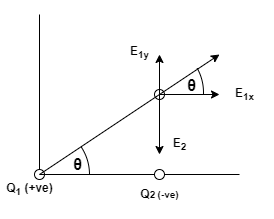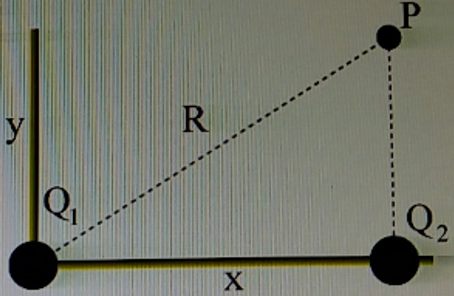# Problem: A positive charge of magnitude Q1 = 9.5 nC is located at the origin. A negative charge Q2 = - 6.5 nC is located on the positive x-axis at x = 3.5 cm from the origin. The point P is located y = 4.5 cm above charge Q2.Part a. Calculate the x-component of the electric field at point P due to charge Q1. Write your answer in units of N/CPart b. Calculate the y-component of the electric field at point P due to the Charge Q1. Write your answer in units of N/CPart c. Calculate the y-component of the electric field at point P due to the Charge Q2. Write your answer in units of N/CPart d. Calculate the y-component of the electric field at point P due to both charges. Write your answer in units of N/C.Part e. Calculate the magnitude of the electric field at point P due to both charges. Write your answer in units of N/CPart f. Calculate the angle in degrees of the electric field at point P relative to the positive x-axis

###### FREE Expert Solution

Electric field:

$\overline{){\mathbf{E}}{\mathbf{=}}\frac{\mathbf{k}\mathbf{q}}{{\mathbf{r}}^{\mathbf{2}}}}$

2D vectors, Magnitude & Direction/Components:

$\overline{)\mathbf{|}\stackrel{\mathbf{⇀}}{\mathbf{E}}\mathbf{|}{\mathbf{=}}\sqrt{{{\mathbf{E}}_{\mathbf{x}}}^{\mathbf{2}}\mathbf{+}{{\mathbf{E}}_{\mathbf{y}}}^{\mathbf{2}}}}$

$\overline{){\mathbf{tan}}{\mathbit{\theta }}{\mathbf{=}}\frac{{\mathbit{E}}_{\mathbit{y}}}{{\mathbit{E}}_{\mathbit{x}}}}$

Part a.

A diagram showing the direction of the vectors and angles will make our work easier.E1x = E1cosθ = kQ1/R2•cosθ

Using Pythagoras theorem:

89% (386 ratings)###### Problem Details

A positive charge of magnitude Q1 = 9.5 nC is located at the origin. A negative charge Q2 = - 6.5 nC is located on the positive x-axis at x = 3.5 cm from the origin. The point P is located y = 4.5 cm above charge Q2.Part a. Calculate the x-component of the electric field at point P due to charge Q1. Write your answer in units of N/C
Part b. Calculate the y-component of the electric field at point P due to the Charge Q1. Write your answer in units of N/C
Part c. Calculate the y-component of the electric field at point P due to the Charge Q2. Write your answer in units of N/C
Part d. Calculate the y-component of the electric field at point P due to both charges. Write your answer in units of N/C.
Part e. Calculate the magnitude of the electric field at point P due to both charges. Write your answer in units of N/C
Part f. Calculate the angle in degrees of the electric field at point P relative to the positive x-axis